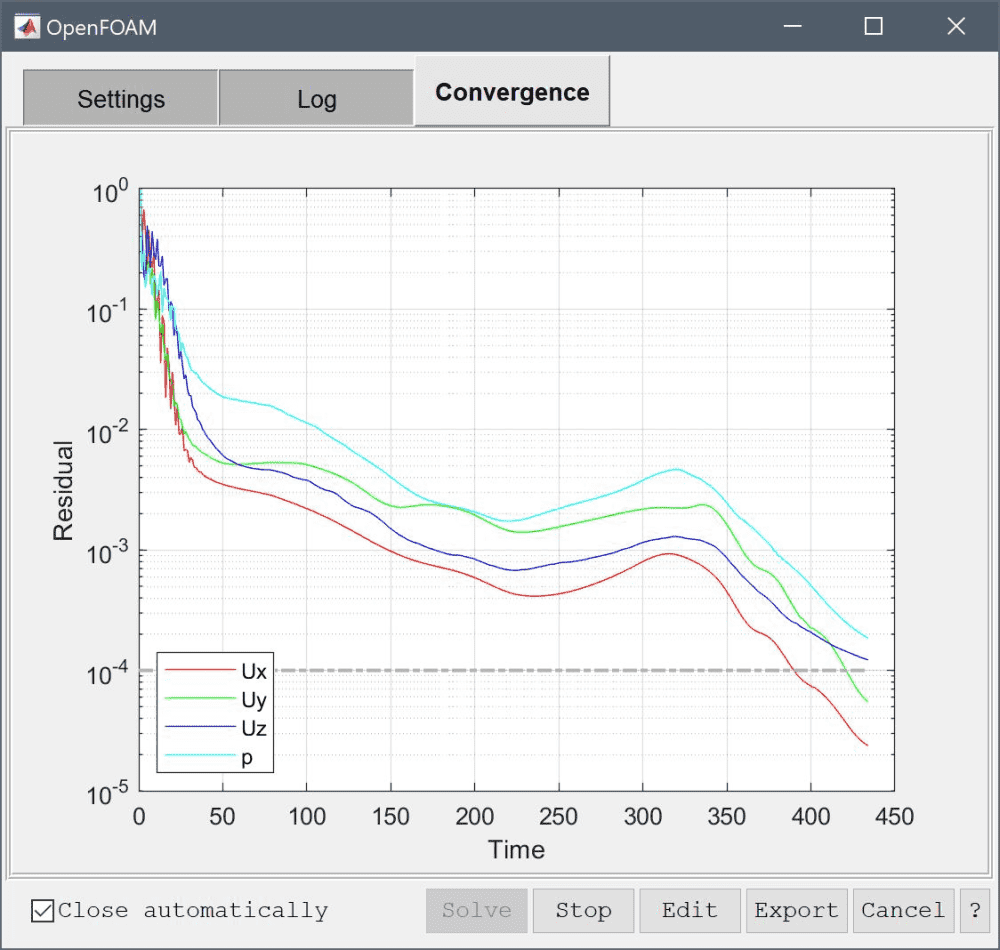# Multi-Simulation Heat ExchangerThis tutorial model illustrates how one can couple the OpenFOAM CFD solver with the FEATool Multiphysics FEA solver. In the model a stream of cool air is heated while flowing through a tube and fin heat exchanger. Due to several symmetry planes only a small section of the full heat exchanger geometry actually needs to be simulated, as illustrated in the following image.The model demonstrates the multi-solver simulation process by first solving for the flow field using the OpenFOAM solver.

After which the temperature field is solved for with the built-in FEATool Multiphysics solver, using the pre-computed flow field as a constant input to the heat equation.

1. Solve flow field with OpenFOAM1. Use the pre-computed velocity field as constant input and solve for the temperature using the built-in multiphysics solver

This two step solution process for a one-way coupled model allows significant savings in computational time and resources, by separating the equations and physics problems, and use the best and most efficient solver for each individual sub-problem.

Although here, the OpenFOAM and built-in solvers are used to demonstrate the multi-simulation process. Other combinations of solvers can also be used such as the FEniCS multiphysics and SU2 solvers.

This model is available as an automated tutorial by selecting Model Examples and Tutorials… > Multiphysics > Multi-Simulation Heat Exchanger from the File menu. Or alternatively, follow the video tutorial or step-by-step instructions linked below.

Tutorial Instructions